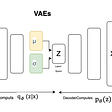# Incorporating element-wise multiplication can out-perform dense layers in neural networks

We ran 1,300 different training experiments to try a large range of different neural network architectures on basic arithmetic, logic and integer tasks. We tested on four different datasets. Surprisingly, the best performing network was one incorporating element-wise multiplication, an infrequently used component in today’s networks. We encourage further experimentation and usage of element-wise multiplication.

In working on MacGraph, a reasoning network that answers questions using knowledge graphs, I came across a problem: which neural network layers should I use to perform the logic and arithmetic functions the dataset requires?

# Background

The type of network that generated this article’s inquiry is a little unusual: whereas most of deep learning is focussed on classifying noisy real world data, MacGraph is focussed on performing multi-step reasoning operations.

By multi-step reasoning, I mean answering questions like “Which newly built station is beside London Bridge?” (from the CLEVR-Graph dataset of mass-transit systems). To answer this sort of question the network needs to be able to extract, filter and combine multiple facts from the available knowledge base.

We often think of neural networks as transforming distributions, or combining many weak signals into strong ones. But in the case of reasoning, the network is often trying to combine pieces of information in a variety of ways (e.g. logical AND, arithmetic counting) depending on the present task and step (in the case of multi-step reasoning RNNs).

In reasoning networks, one can think of three distinct phases of the network (these may be multiple iterations of an RNN, or multiple layers in a FNN):

Depending on the question, each reasoning operation may need to do the same or different calculation. For the CLEVR dataset, successful models need to combine filtering, AND, OR, existence, counting, equality, uniqueness and relational operations to mimic the functional program used to generate the answer label.

Here’s the description given by the authors of CLEVR:

Therefore, for capable reasoning systems, it’s vital that the reasoning operation cells can perform many/all of the aforementioned operations.

This article focuses on the question “Which neural network components should form the reasoning operation?”. Here we will focus on implementing the individual types of reasoning operations, and leave discussion of how to conditionally combine them depending on the question for a future work.

# Experiment

To find out which neural network components are best at reasoning operations, we’ve put together a set of reasoning operation tasks, a set of datasets and a set of different neural network components. We’ll test all combinations of them to see which achieve the greatest test accuracy.

The networks were tested on a wide range of tasks. These represent many of the things a reasoning network needs to do to solve CLEVR and other similar challenges.

• Vector concatenation
• Elementwise multiplication
• Vector dot product
• Reduce-sum (e.g. add every element together)
• Equality between vectors
• Integer addition (where integers are represented as one-hot vectors)
• Logical AND, OR and XOR

## Datasets

Our datasets each contain one million training records and four thousand evaluation records. Each record contains two vectors as the input features, and an output vector as the label. Input vectors are width 128, output vectors width depends on the task being tested.

Each training and evaluation record (e.g. pair of vectors) is unique, there is no overlap between the two sets.

Typically networks are allowed to learn their own internal representation of information (either through embeddings or feed-forward transformations). Depending on the nature and constraints of the network, these representations may have different characteristics.

For example, if a sigmoid activation is used, the internal representation will have only positive elements between 0.0 and 1.0. Or, if Word2Vec word embeddings are used, vectors look like strings of random floating point numbers.

To try and simulate the different possibilities in real networks, the following datasets have been generated:

• One hot vectors
• Many hot vectors
• Random vectors between -1.0 and 1.0
• Random positive vectors between 0.0 and 1.0

# Networks tested

A wide range of popular networks were tested.

## Dense and Residual

Single, double and triple layer dense network stacks were tested. Hidden layers had the width of the combined input features, i.e. 256 elements. The output layer had the width the task dictated (e.g. 128 wide for element-wise addition)

A range of activation functions were tested, representative of popular configurations in the real world:

• No activation (e.g. pure linear)
• SELU
• ReLU
• Sigmoid
• Tanh

Both plain dense layer stacks and residual stacks were tested. In the residual stacks, every time the input and output width of a layer were the same (e.g. all layers apart from the output) a residual “skip” connection was added where the layer’s input was added to the layer’s output.

## Multiply unit

For comparison, a multiply unit was also tested. This consisted of applying linear dense layers to each input (each hidden width 256), then performing element-wise multiplication, then applying final linear dense layer (output width as dictated by the task):

A second multiply cell, “multiply simple” was also tested. It is the same as above, but without the linear dense layers on the inputs (i.e. it is elementwise multiplication followed by a single linear dense layer).

# Experimental procedure

Each combination of task, network and dataset was tested. Some combinations are trivial (e.g. reduce sum of two one hot vectors always gives 2.0) and some don’t make sense (e.g. logical operations on random floating point numbers) and these have been excluded from the results analysis.

Each combination was first grid-searched to find the best learning rate (e.g. learning rates from 0.000001 to 100.0 were tried for 1,000 training steps, with the lowest loss training’s learning rate then used to train the network from scratch to 30,000 training steps. Early stopping was used for any time a network achieved 100% accuracy).

The Adam optimizer was used and mean-squared error was used to calculate the loss of the network’s output compared to the desired label.

# Results

Few networks were able to succeed on the majority of the tasks and datasets tested. Here’s the high level summary of how each network performed:

Surprisingly, the multiply network achieved the best success score, despite being an unusual network structure. The residual networks performed worst, despite being incredibly popular in deep visual networks due to their flexibility. Let’s dig into the details.

## The multiply network performed perfectly on almost all tasks

As you can see above, the multiply network achieved close to 100% on almost every task on every dataset.

Given this result, we suggest that multiplication operations may be an overlooked ingredient to designing reasoning and general neural networks

Notably, the multiply network consistently struggled with two tasks: dot product and reduce sum.

It was surprising that the multiply network struggled on the dot product task as the two are structurally similar. We suspected that this failure could be because the back-propagation is under-constrained: the network outputs a single scalar and from its error has to optimize three linear dense layers (i.e. 66k parameters). To test this hypothesis, we removed the two initial dense layers to create the “multiply simple” network and tested it:

The multiply simple network achieved 100% on all tasks, confirming that the two initial linear dense layers were indeed the problem.

Neither variation did well on the reduce sum task (the one hot dataset was excluded as it’s answer for this task is always 2.0):

For the reduce sum task, the network needs to preserve both input vectors across the element-wise multiplication, in order to sum their elements in the final dense layer. Achieving that by manipulating the inputs prior to multiplication is an intricate operation and one the network failed to learn. Understandably, the multiply simple network completely failed as its inputs were irrecoverably corrupted by the element-wise multiplication.

## Most networks performed very well on one-hot vectors

There was something that all the network variants performed very well on: one-hot vectors.

One-hot vectors are the simplest to process. Generally operations have fewer possible cases to consider compared to many hot or random vectors. Some operations become trivial as their output is always the same (e.g. reduce sum).

As you can see in the table below, pretty much all networks got >99% accuracy on most tasks: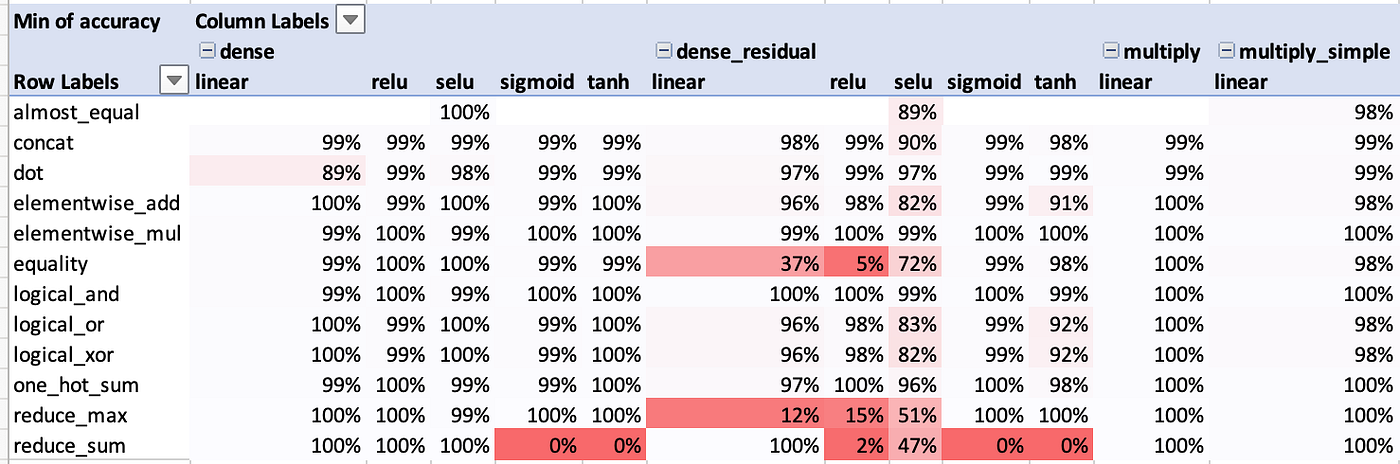This table shows the worst performance for the dense networks amongst the 1, 2 and 3 layer variations

There were a couple of common failure modes:

## Activation functions’ range must include the output domain

A really simple cause of some of the failures was because the activation function (e.g. sigmoid) had a range (0.0 to 1.0) that did not include the output label domain for the network (e.g. reduce sum’s domain is all real numbers). This affected sigmoid, tanh, ReLU and SELU.

## Residual networks performed worse than non-residual

Something to do with unavoidably mixing in the previous distribution with the new one?

## Logical XOR was the hardest logical task for the networks

All networks performed worse on logical XOR than on AND or OR. Below shows the networks performance on many hot vectors (the hardest dataset for logical tasks):

As mentioned earlier, multiply performs best with dense, dense residual and multiply simple following afterwards.

## Network performance was very sensitive to number of layers and activation function

One of the hard things in putting together this analysis was the sheer irregularity of networks’ performance. The table below shows the whole picture:

Having too many or too few layers impeded most networks’ performance, switching activation functions could drastically alter their ability to train to a high accuracy. Furthermore, a network that excels at one task often fails badly on some others.

This gives the difficult result that there may not be many easy “one size fits all” answers for this area of engineering. Multiplication networks performed remarkably well, but there remains more work to create a composite (perhaps ensemble) approach that performs best in all scenarios.

# Conclusions

We’ve shown the performance of 23 networks on 10 tasks against 4 datasets, internally running 1,300 different experiments and 11,700 separate trainings.

Multiplication based networks achieved the highest performance, despite being a relatively unknown ingredient in building neural networks. We propose that they warrant further experimentation and consideration in a research engineer’s toolkit.

## Octavian’s research

Octavian’s mission is to develop systems with human-level reasoning capabilities. We believe that graph data and deep learning are key ingredients to making this possible. If you interested in learning more about our research or contributing, check out our website or get in touch.

## Appendix

Here is a full listing of the networks individual performance:

--

--

--

## More from Octavian

Research into machine learning and reasoning

## A brief history of Neural Networks.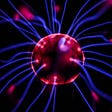## G2D: Generate to Detect Anomaly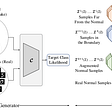## How to Estimate ROI and Costs for Machine Learning and Data Science Projects## SVD — A geometric view point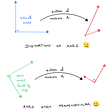## How to get started with machine learning on graphs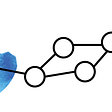## GAN weekly update, week #27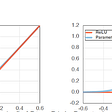## Machine Learning Pipeline: Architecture of ML Platform in Production## Continuous Delivery for Machine Learning Systems## David Mack

@SketchDeck co-founder, https://octavian.ai researcher, I enjoy exploring and creating.

## Predicting ride conversion rate with Keras/Tensorflow (Part I — Building the network)## Light Survey about Transformer Model — Part I## Breakdown and Utilization of a Convolutional Neural Network## Deep Generative Modeling(DGM)